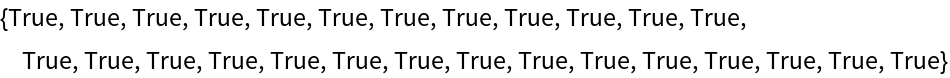#Function Repository Resource:

# AnagramQ

Test whether strings are anagrams

Contributed by: Sander Huisman
 ResourceFunction["AnagramQ"][s1,s2] yields True if s1 is an anagram of s2. ResourceFunction["AnagramQ"][s1,s2,…] yields True if all the strings si are anagrams of one another.

## Details and Options

By default, spaces and the case of the letters are ignored.
ResourceFunction["AnagramQ"] has the following options:
 treats lowercase and uppercase letters as equivalent. treats diacritics and regular letters as equivalent. "IgnoreSpaces" ignores spaces from the strings.
The option IgnoreDiacritics True effectively applies RemoveDiacritics to each string before the comparison. The outcome of RemoveDiacritics might depend on the \$Language setting.
ResourceFunction["AnagramQ"] has an arbitary arity.
Like Equal and SameQ, ResourceFunction["AnagramQ"] without any arguments (i.e. ResourceFunction["AnagramQ"][]) yields True.
Like Equal and SameQ, ResourceFunction["AnagramQ"] with a single argument (i.e. ResourceFunction["AnagramQ"][s1]) yields True.
ResourceFunction["AnagramQ"] with more than two arguments will check if all the arguments are anagrams of each other.

## Examples

### Basic Examples

Test whether “trace” is an anagram of “crate”:

 In:=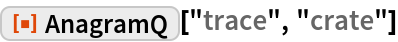Out=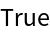Test whether “the Morse code” is an anagram of “Here come dots”:

 In:=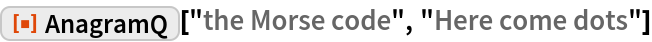Out=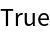### Scope

AnagramQ accepts more than two arguments and will check if all of them are anagrams of one another:

 In:=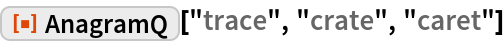Out=### Options

Use the option IgnoreCase to ignore the case of the letters:

 In:=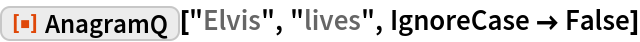Out=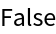In:=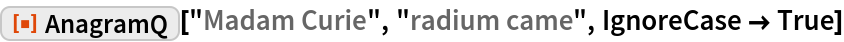Out=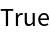Use the option IgnoreDiacritics to ignore diacritics:

 In:=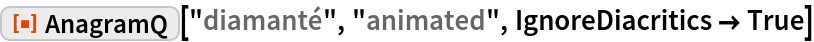Out=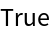In:=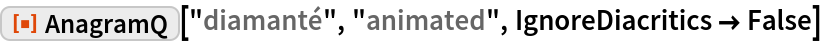Out=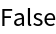Use the option "IgnoreSpaces" to decide whether or not to ignore spaces:

 In:=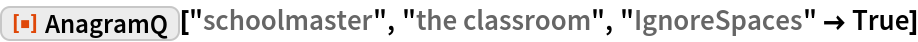Out=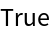In:=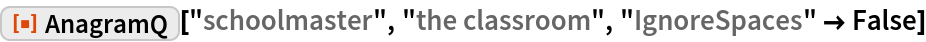Out=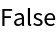### Applications

Look for anagrams of a word using the built-in dictionary:

 In:=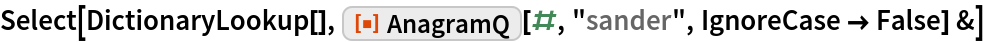Out=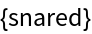Test whether two sentences are anagrams of one another:

 In:=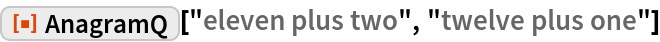Out=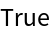### Possible Issues

Two equal strings are not considered anagrams:

 In:=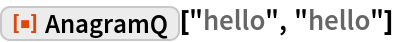Out=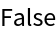Two empty strings are also not considered anagrams of one another:

 In:=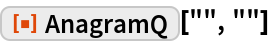Out=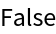AnagramQ returns True if a single argument is supplied:

 In:=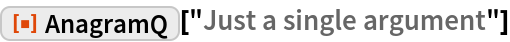Out=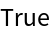AnagramQ returns True if no arguments are supplied:

 In:=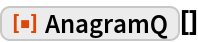Out=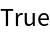Depending on \$Language the option IgnoreDiacritics can affect the outcome:

 In:=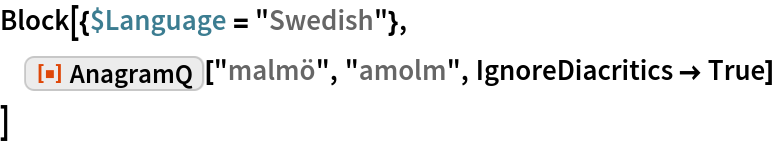Out=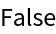In:=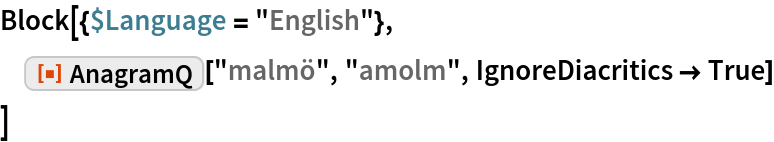Out=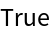### Neat Examples

Find anagrams with six or more words with at least five letters:

 In:=Out=Confirm that all the groups of words are anagrams:

 In:=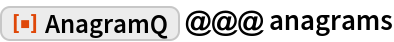Out=